# The theory of Antenna Radiation

Antennas are everywhere. Whether it be in the form of large towers sitting atop rooftops on buildings, satellite dishes, or even in the form of very tiny printed circuits on your smartphone; antennas play a crucial role in transmitting information across the globe and into outer space without the need for wires or cables spanning ridiculous distances. So how does an antenna work, exactly? To answer this question, we will look at how electromagnetic waves can be produced in a simple model known as the Hertzian dipole antenna.

*Note: this article assumes you are familiar with some vector calculus and with Maxwell’s equations. If you would like a more foundational look at these, you may check out my other articles on topics such as “Deriving Gauss’s law from Coulomb’s law” and “Deriving Gauss’s law of magnetism”

# The equations that describe antenna radiation in general

Antenna radiation can be studied in the context of electromagnetic (EM) waves using Maxwell’s equations in frequency-space, namely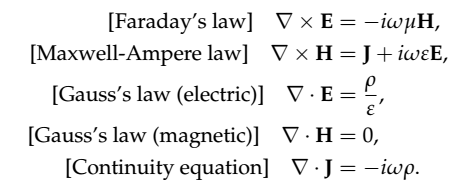Equation 1 (a) - (e)

These equations represent the electric field E and magnetizing field H (not to be confused with the magnetic field B, although in special cases these two are related by the equation B =𝜇H), along with a current density J and a charge density 𝜌. The constants 𝜇 and 𝜀 represent the permeability and permittivity of the propagation medium, respectively. The parameter 𝜔 represents an arbitrary frequency of oscillation (in units of rad/s, related to the frequency f in Hertz via 𝜔 = 2𝜋f), which can take on multiple values since waves can be constructed by adding multiple waves of different frequencies and amplitudes, so that the electric and magnetic fields are functions of space and frequency. This version of Maxwell’s equations is valid when we assume the EM fields to be time-harmonic (e.g. described by a series of sinusoids in time), and are obtained by taking the Fourier transform of the time-dependent equations (for instance, the Fourier transform of a time-varying quantity x(t) is Fourier[x(t)] = i𝜔X(𝜔), where i is the imaginary unit defined as i² = -1).

To get a solution to these equations, we focus on Eq(1a) first, by taking the curl of both sides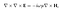Equation 2

and substituting the left hand side by

by using what is known as the “double-curl identity of vector calculus”. Next, we replace the right-hand side of Eq(2) by Eq(1b) to obtain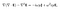Equation 3

which can be re-arranged into

At this point, we can make the substitution

such that we end up with an equation for the electric field E explicitly in terms of the current density J only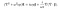Equation 4: electric field as a function of current density

Using the method of Green’s functions, we can solve this equation in terms of the following operator corresponding to Helmholtz-type equations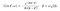Equation 5: Green’s function for the Helmholtz-type equation

thus integrating both sides of Eq(4) with respect to r’ yields the solution to the electric fieldEquation 6: solution for the electric field as a function of the current density J

Note that this equation works for any type of current density J, by evaluating the integral

and from this we calculate the magnetizing field as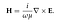Equation 7: magnetizing field as a function of the electric field

However, a more enlightening result is derived if we take the curl of both sides of Eq(6), which yields

the 2nd term becomes zero because of the vector identity

Then, we can write the magnetizing field as

and if we now define the vector potential A as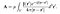Equation 8

we can write both electric and magnetizing fields as functions of this vector potential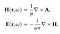Equation 9 (a) - (b)

# Approximate analysis of a dipole antenna

Eq(9a) and (9b) cannot be solved explicitly in most cases due to the complexity of the potential A in Eq(8). We can, however, consider a very simple case in which we possess a straight filament of length L oriented along the z-axis with current I, for which the current density may be written as

over the range

where 𝛥A is the cross-section of the rod, as shown in Figure 1(a).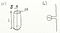Figure 1: (a) conducting wire with cross-section 𝛥A, (b) simple dipole antenna with a sinusoidal source

This can be easily generated by connecting two L-shaped conducting rods to a voltage source, creating a filament of length L as in Figure 1(b) . The idea is to predict the radiation pattern for the case where L<<𝜆, where 𝜆 is the wavelength of the source. Furthermore, we assume that the width of the antenna itself is very small comparable to its length, such that we can write

Also, the length of the antenna is assumed to be very small compared to the distance in the far-field measurement, such that the displacement vector r - r’ does not vary much along z and can be treated as a constant r inside the integral. Introducing this expression into the integral in Eq(8) yields the vector potential in

However, since we expect the radiation pattern to be better represented in spherical coordinates, we now convert this expression into spherical coordinates via the matrix equation

Given that

we directly

Next, we take the curl of the field

from which we calculate the magnetizing field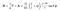Equation 10: magnetizing field for a dipole source

Next we calculate the electric field outside the region of charge

and taking the curl of H we obtain

which yields the expression for the electric fieldEquation 11: electric field for a dipole source

# Near-field and far-field approximations

Eq(10) and Eq(11) are very complicated to understand due to the multiple terms they have. For this reason, it is often useful to look at two regions of interest. Firstly, we look at the near-field case where r<<𝜆, where the term r^(-n) with the highest n dominates over all others, which allows us to write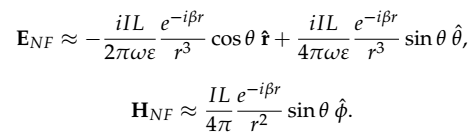Equation 12 (a) - (b): near-field approximations

Similarly, we can write equations for the far-field case in which r>>𝜆 such that all terms r^(-n) for n > 0 are neglected, yielding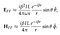Equation 13 (a) - (b): far-field approximations

Oscar is a physicist, educator and STEM enthusiast. He is currently finishing a PhD in Theoretical Physics with a focus on photonics and stochastic dynamics.

## More from Oscar Nieves

Oscar is a physicist, educator and STEM enthusiast. He is currently finishing a PhD in Theoretical Physics with a focus on photonics and stochastic dynamics.